• RNN循环神经网络结构 note: RNN处理有序的数据。例如一句话 一层双向的循环神经网络示意图（没有 S0' 则为单层循环）: 正向循环S0 到 Si：y1的值受X1,A1以及上一层的A0影响 反向循环Si 到 S0：y1...


RNN循环神经网络结构

note: RNN处理有序的数据。例如一句话

一层双向的循环神经网络示意图（没有 S0' 则为单层循环）:
正向循环S0 到 Si：y1的值受X1,A1以及上一层的A0影响
反向循环Si 到 S0：y1的值受X1, A1'  以及下一层的 A2'  影响
他们两者之间并不共享权值。
多层双向循环构成深度循环神经网络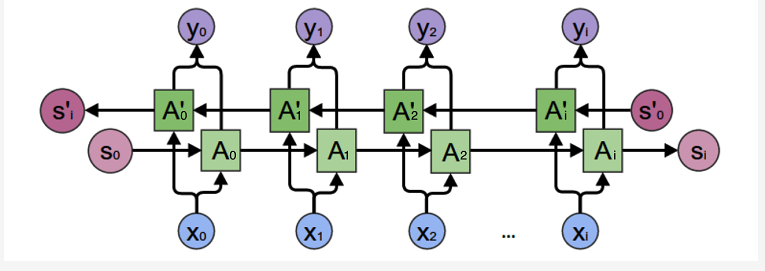posted on 2018-06-25 13:49 .Tang 阅读(...) 评论(...)  编辑 收藏

转载于:https://www.cnblogs.com/tangpg/p/9223981.html
展开全文• 几种常见的循环神经网络结构RNN、LSTM、GRU 一、循环神经网络(RNN) 传统文本处理任务的方法中一般将TF-IDF向量作为特征输入。显而易见，这样的表示实际上丢失了输入的文本序列中每个单词的顺序。在神经网络的建模...
几种常见的循环神经网络结构RNN、LSTM、GRU

一、循环神经网络(RNN)

传统文本处理任务的方法中一般将TF-IDF向量作为特征输入。显而易见，这样的表示实际上丢失了输入的文本序列中每个单词的顺序。在神经网络的建模过程中，一般的前馈神经网络，如卷积神经网络，通常接受一个定长的向量作为输入。卷积神经网络对文本数据建模时，输入变长的字符串或者单词串，然后通过滑动窗口加池化的方式将原先的输入转换成一个固定长度的向量表示，这样做可以捕捉到原文本中的一些局部特征，但是两个单词之间的长距离依赖关系还是很难被学习到。
循环神经网络却能很好地处理文本数据变长并且有序的输入序列。它模拟了人阅读一篇文章的顺序，从前到后阅读文章中的每一个单词，将前面阅读到的有用信息编码到状态变量中去，从而拥有了一定的记忆能力，可以更好地理解之后的文本。
其网络结构如下图所示：循环神经网络

由图可见，t是时刻，x是输入层，s是隐藏层，o是输出层，矩阵W就是隐藏层上一次的值作为这一次的输入的权重。输出层o和隐藏层s的计算方法

如果反复把式 2 带入到式 1，将得到：反复把式2带入到式1，得到的形式如图

其中f和g为激活函数，U为输入层到隐含层的权重矩阵，W为隐含层从上一时刻到下一时刻状态转移的权重矩阵。在文本分类任务中，f可以选取Tanh函数或者ReLU函数，g可以采用Softmax函数。

通过最小化损失误差（即输出的y与真实类别之间的距离），我们可以不断训练网络，使得得到的循环神经网络可以准确地预测文本所属的类别，达到分类目的。相比于卷积神经网络等前馈神经网络，循环神经网络由于具备对序列顺序信息的刻画能力，往往能得到更准确的结果。

训练算法

RNN的训练算法为：BPTT
BPTT的基本原理和BP算法是一样的，同样是三步：
1.前向计算每个神经元的输出值；
2.反向计算每个神经元的误差项值，它是误差函数E对神经元j的加权输入的偏导数；
3.计算每个权重的梯度。
最后再用随机梯度下降算法更新权重。
具体参考：https://www.jianshu.com/p/39a99c88a565
最后由链式法则得到下面以雅可比矩阵来表达的每个权重的梯度:循环层权重矩阵W的梯度的公式循环层权重矩阵U的梯度的公式

由于预测的误差是沿着神经网络的每一层反向传播的，因此当雅克比矩阵的最大特征值大于1时，随着离输出越来越远，每层的梯度大小会呈指数增长，导致梯度爆炸；反之，若雅克比矩阵的最大特征值小于1，梯度的大小会呈指数缩小，产生梯度消失。对于普通的前馈网络来说，梯度消失意味着无法通过加深网络层次来改善神经网络的预测效果，因为无论如何加深网络，只有靠近输出的若干层才真正起到学习的作用。这使得循环神经网络模型很难学习到输入序列中的长距离依赖关系。

梯度爆炸的问题可以通过梯度裁剪来缓解，即当梯度的范式大于某个给定值时，对梯度进行等比收缩。而梯度消失问题相对比较棘手，需要对模型本身进行改进。深度残差网络是对前馈神经网络的改进，通过残差学习的方式缓解了梯度消失的现象，从而使得我们能够学习到更深层的网络表示；而对于循环神经网络来说，长短时记忆模型及其变种门控循环单元等模型通过加入门控机制，很大程度上弥补了梯度消失所带来的损失。

二、长短期记忆网络(LSTM)

LSTM的网络机构图如下所示：LSTM的网络结构

与传统的循环神经网络相比，LSTM仍然是基于xt和ht−1来计算ht，只不过对内部的结构进行了更加精心的设计，加入了输入门it 、遗忘门ft以及输出门ot三个门和一个内部记忆单元ct。输入门控制当前计算的新状态以多大程度更新到记忆单元中；遗忘门控制前一步记忆单元中的信息有多大程度被遗忘掉；输出门控制当前的输出有多大程度上取决于当前的记忆单元。

在经典的LSTM模型中，第t层的更新计算公式为输入门计算公式遗忘门计算公式输出门计算公式候选层计算公式记忆单元更新公式每层输出的信息的计算公式

其中it是通过输入xt和上一步的隐含层输出ht−1进行线性变换，再经过激活函数σ得到的。输入门it的结果是向量，其中每个元素是0到1之间的实数，用于控制各维度流过阀门的信息量；Wi 、Ui两个矩阵和向量bi为输入门的参数，是在训练过程中需要学习得到的。遗忘门ft和输出门ot的计算方式与输入门类似，它们有各自的参数W、U和b。与传统的循环神经网络不同的是，从上一个记忆单元的状态ct−1到当前的状态ct的转移不一定完全取决于激活函数计算得到的状态，还由输入门和遗忘门来共同控制。

在一个训练好的网络中，当输入的序列中没有重要信息时，LSTM的遗忘门的值接近于1，输入门的值接近于0，此时过去的记忆会被保存，从而实现了长期记忆功能；当输入的序列中出现了重要的信息时，LSTM应当把其存入记忆中，此时其输入门的值会接近于1；当输入的序列中出现了重要信息，且该信息意味着之前的记忆不再重要时，输入门的值接近1，而遗忘门的值接近于0，这样旧的记忆被遗忘，新的重要信息被记忆。经过这样的设计，整个网络更容易学习到序列之间的长期依赖。

三、门控循环单元(GRU)

GRU是在LSTM上进行简化而得到的，GRU的网络结构如下所示：GRU的网络结构图

更新门的作用类似于LSTM中的遗忘门和输入门，它能决定要丢弃哪些信息和要添加哪些新信息。

重置门用于决定丢弃先前信息的程度。

四、参考资料

1.百面机器学习
2.https://zhuanlan.zhihu.com/p/45649187
3.https://www.jianshu.com/p/39a99c88a565

作者：Evermemo
链接：https://www.jianshu.com/p/0cf7436c33ae
來源：简书
简书著作权归作者所有，任何形式的转载都请联系作者获得授权并注明出处。
展开全文• 循环神经网络让神经网络有了记忆, 对于序列话的数据,循环神经网络能达到更好的效果.上次我们提到了用 RNN 的最后一个时间点输出来判断之前看到的图片属于哪一类, 这次我们来真的了, 用 RNN 来及时预测时间序列. ...
目录

1.写在前面

2.训练数据

3.RNN模型

4.训练

5.完整代码演示

1.写在前面

循环神经网络让神经网络有了记忆, 对于序列话的数据,循环神经网络能达到更好的效果.上次我们提到了用 RNN 的最后一个时间点输出来判断之前看到的图片属于哪一类, 这次我们来真的了, 用 RNN 来及时预测时间序列.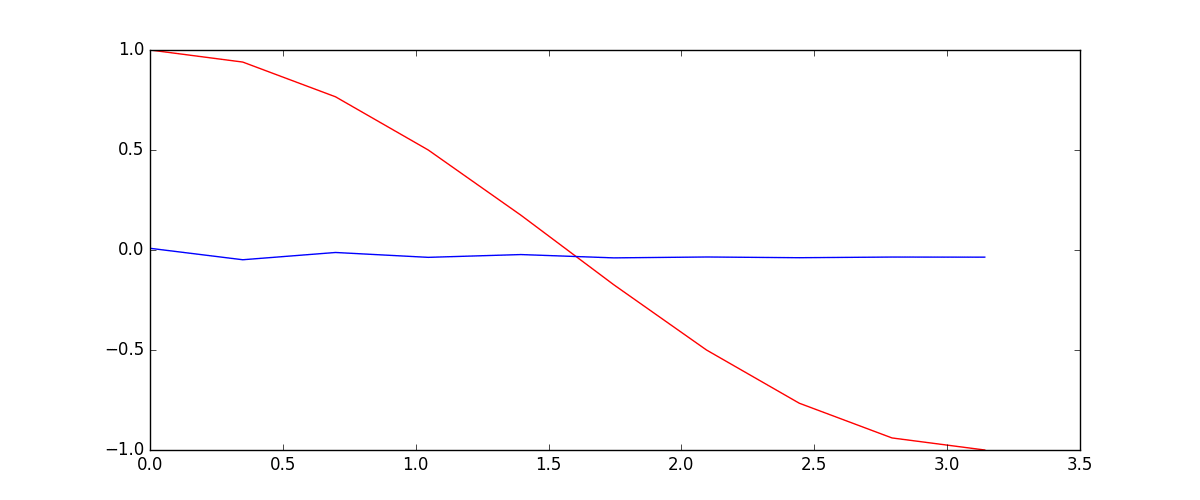2.训练数据

我们要用到的数据就是这样的一些数据, 我们想要用 sin 的曲线预测出 cos 的曲线.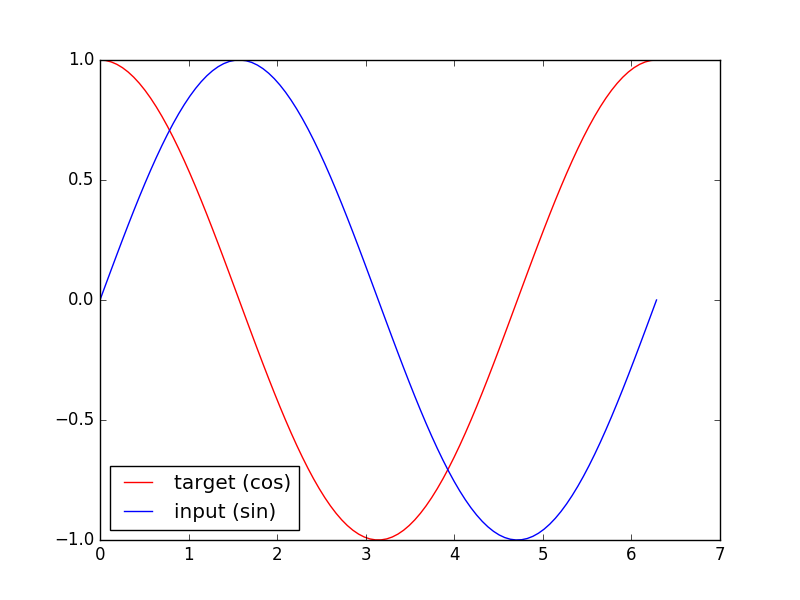import torch
from torch import nn
import numpy as np
import matplotlib.pyplot as plt

torch.manual_seed(1)    # reproducible

# Hyper Parameters
TIME_STEP = 10      # rnn time step / image height
INPUT_SIZE = 1      # rnn input size / image width
LR = 0.02           # learning rate
DOWNLOAD_MNIST = False  # set to True if haven't download the data

3.RNN模型

这一次的 RNN, 我们对每一个 r_out 都得放到 Linear 中去计算出预测的 output, 所以我们能用一个 for loop 来循环计算. 这点是 Tensorflow 望尘莫及的! 看看我们的 PyTorch 和 Tensorflow 到底哪家强.

class RNN(nn.Module):
def __init__(self):
super(RNN, self).__init__()

self.rnn = nn.RNN(  # 这回一个普通的 RNN 就能胜任
input_size=1,
hidden_size=32,     # rnn hidden unit
num_layers=1,       # 有几层 RNN layers
batch_first=True,   # input & output 会是以 batch size 为第一维度的特征集 e.g. (batch, time_step, input_size)
)
self.out = nn.Linear(32, 1)

def forward(self, x, h_state):  # 因为 hidden state 是连续的, 所以我们要一直传递这一个 state
# x (batch, time_step, input_size)
# h_state (n_layers, batch, hidden_size)
# r_out (batch, time_step, output_size)
r_out, h_state = self.rnn(x, h_state)   # h_state 也要作为 RNN 的一个输入

outs = []    # 保存所有时间点的预测值
for time_step in range(r_out.size(1)):    # 对每一个时间点计算 output
outs.append(self.out(r_out[:, time_step, :]))
return torch.stack(outs, dim=1), h_state

rnn = RNN()
print(rnn)
"""
RNN (
(rnn): RNN(1, 32, batch_first=True)
(out): Linear (32 -> 1)
)
"""

其实熟悉 RNN 的朋友应该知道, forward 过程中的对每个时间点求输出还有一招使得计算量比较小的. 不过上面的内容主要是为了呈现 PyTorch 在动态构图上的优势, 所以我用了一个 for loop 来搭建那套输出系统. 下面介绍一个替换方式. 使用 reshape 的方式整批计算.

def forward(self, x, h_state):
r_out, h_state = self.rnn(x, h_state)
r_out = r_out.view(-1, 32)
outs = self.out(r_out)
return outs.view(-1, 32, TIME_STEP), h_state

4.训练

下面的代码就能实现动图的效果啦~开心, 可以看出, 我们使用 x 作为输入的 sin 值, 然后 y 作为想要拟合的输出, cos 值. 因为他们两条曲线是存在某种关系的, 所以我们就能用 sin 来预测 cos. rnn 会理解他们的关系, 并用里面的参数分析出来这个时刻 sin 曲线上的点如何对应上 cos 曲线上的点.optimizer = torch.optim.Adam(rnn.parameters(), lr=LR)   # optimize all rnn parameters
loss_func = nn.MSELoss()

h_state = None   # 要使用初始 hidden state, 可以设成 None

for step in range(100):
start, end = step * np.pi, (step+1)*np.pi   # time steps
# sin 预测 cos
steps = np.linspace(start, end, 10, dtype=np.float32)
x_np = np.sin(steps)    # float32 for converting torch FloatTensor
y_np = np.cos(steps)

x = torch.from_numpy(x_np[np.newaxis, :, np.newaxis])    # shape (batch, time_step, input_size)
y = torch.from_numpy(y_np[np.newaxis, :, np.newaxis])

prediction, h_state = rnn(x, h_state)   # rnn 对于每个 step 的 prediction, 还有最后一个 step 的 h_state
# !!  下一步十分重要 !!
h_state = h_state.data  # 要把 h_state 重新包装一下才能放入下一个 iteration, 不然会报错

loss = loss_func(prediction, y)     # cross entropy loss
optimizer.zero_grad()               # clear gradients for this training step
loss.backward()                     # backpropagation, compute gradients
optimizer.step()                    # apply gradients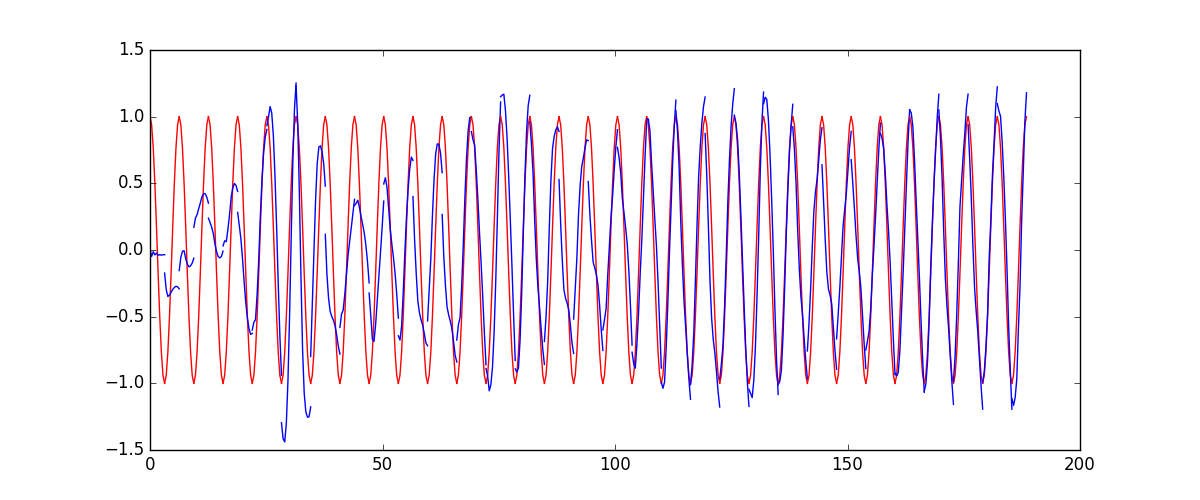5.完整代码演示

import torch
from torch import nn
import numpy as np
import matplotlib.pyplot as plt

# torch.manual_seed(1)    # reproducible

# Hyper Parameters
TIME_STEP = 10      # rnn time step
INPUT_SIZE = 1      # rnn input size
LR = 0.02           # learning rate

# show data
steps = np.linspace(0, np.pi*2, 100, dtype=np.float32)  # float32 for converting torch FloatTensor
x_np = np.sin(steps)
y_np = np.cos(steps)
plt.plot(steps, y_np, 'r-', label='target (cos)')
plt.plot(steps, x_np, 'b-', label='input (sin)')
plt.legend(loc='best')
plt.show()

class RNN(nn.Module):
def __init__(self):
super(RNN, self).__init__()

self.rnn = nn.RNN(
input_size=INPUT_SIZE,
hidden_size=32,     # rnn hidden unit
num_layers=1,       # number of rnn layer
batch_first=True,   # input & output will has batch size as 1s dimension. e.g. (batch, time_step, input_size)
)
self.out = nn.Linear(32, 1)

def forward(self, x, h_state):
# x (batch, time_step, input_size)
# h_state (n_layers, batch, hidden_size)
# r_out (batch, time_step, hidden_size)
r_out, h_state = self.rnn(x, h_state)

outs = []    # save all predictions
for time_step in range(r_out.size(1)):    # calculate output for each time step
outs.append(self.out(r_out[:, time_step, :]))
return torch.stack(outs, dim=1), h_state

# instead, for simplicity, you can replace above codes by follows
# r_out = r_out.view(-1, 32)
# outs = self.out(r_out)
# outs = outs.view(-1, TIME_STEP, 1)
# return outs, h_state

# or even simpler, since nn.Linear can accept inputs of any dimension
# and returns outputs with same dimension except for the last
# outs = self.out(r_out)
# return outs

rnn = RNN()
print(rnn)

optimizer = torch.optim.Adam(rnn.parameters(), lr=LR)   # optimize all cnn parameters
loss_func = nn.MSELoss()

h_state = None      # for initial hidden state

plt.figure(1, figsize=(12, 5))
plt.ion()           # continuously plot

for step in range(100):
start, end = step * np.pi, (step+1)*np.pi   # time range
# use sin predicts cos
steps = np.linspace(start, end, TIME_STEP, dtype=np.float32, endpoint=False)  # float32 for converting torch FloatTensor
x_np = np.sin(steps)
y_np = np.cos(steps)

x = torch.from_numpy(x_np[np.newaxis, :, np.newaxis])    # shape (batch, time_step, input_size)
y = torch.from_numpy(y_np[np.newaxis, :, np.newaxis])

prediction, h_state = rnn(x, h_state)   # rnn output
# !! next step is important !!
h_state = h_state.data        # repack the hidden state, break the connection from last iteration

loss = loss_func(prediction, y)         # calculate loss
optimizer.zero_grad()                   # clear gradients for this training step
loss.backward()                         # backpropagation, compute gradients
optimizer.step()                        # apply gradients

# plotting
plt.plot(steps, y_np.flatten(), 'r-')
plt.plot(steps, prediction.data.numpy().flatten(), 'b-')
plt.draw(); plt.pause(0.05)

plt.ioff()
plt.show()


展开全文• 循环神经网络结构简介
本文只是简单的说明一下RNN的结构，不涉及RNN的发展历史
一般的神经网络，比如前馈神经网络，其特点是：
1  共有输入层，隐层，输出层3层；
2  输入层的输入与时间并无关系
隐层的每一层的神经元彼此之间并不相连
输出层的输出是由所有的输入层以及网络架构决定的，与时间无关
而循环神经网络恰恰相反，即：
1   隐层之间的节点不是相互独立而是有连接的，且隐层的输入不仅包括输入层的输出，还包括上一时刻隐藏层的输出
2   而且输入层进行输入后，而是随时间进行变化进行相应的计算，同时每次计算都会产生一个输出，即就是输出也是随时间变化的
二者对比如下：左边为一个简单的神经网络结构图，右边则为RNN结构图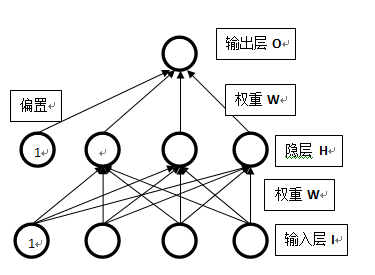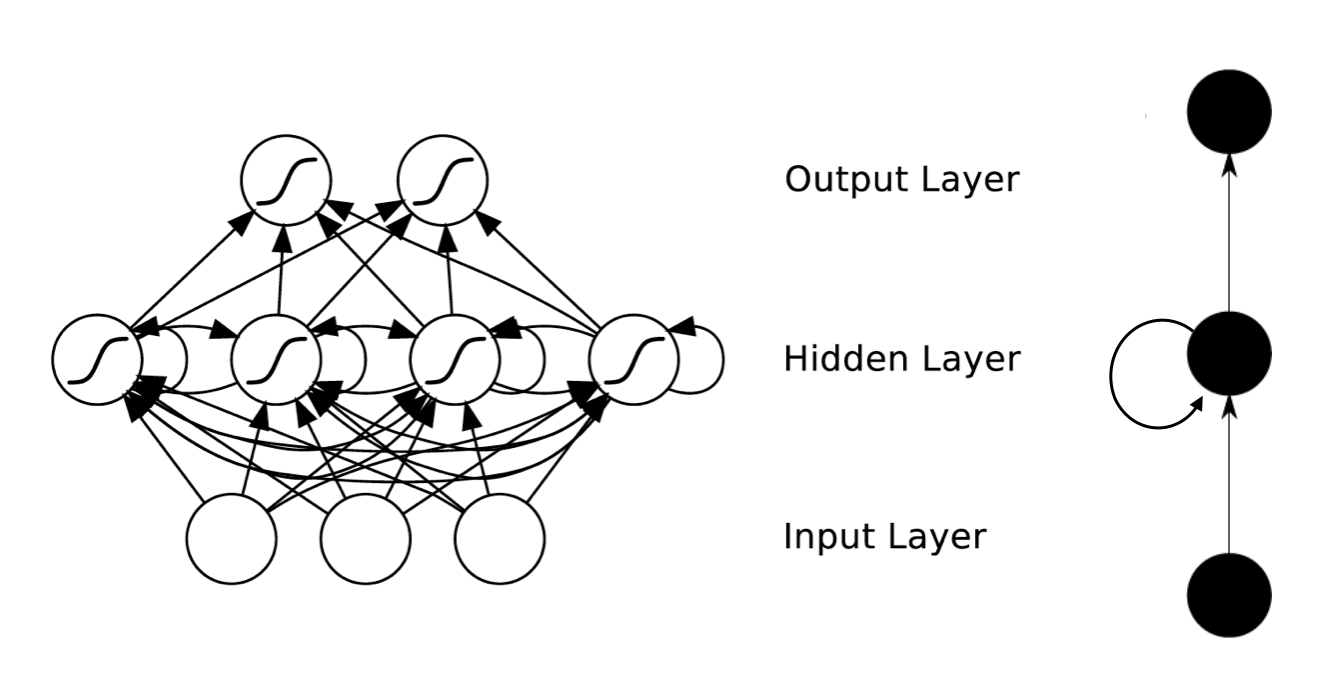简单的神经网络                                                                                                                      循环神经网络
看起来循环神经网络很复杂，我们可以循环神经网络进行展开：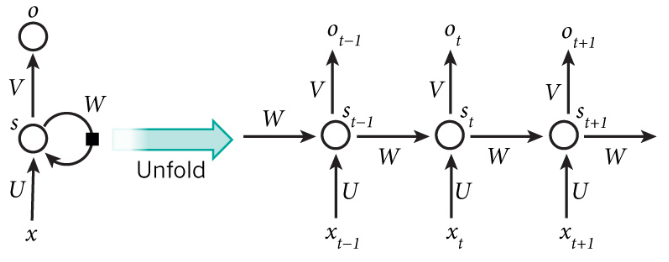参数意义：o表示输出，x为输入，s为隐层，U,V,W均为权重
那么，了解了RNN的结构，我们还需要知道怎么进行操作：
如上图所示，令X = {x1,x2,x3,......xt,xt+1,......}表示输入；令 O={o1,o2,.......ot,ot+1,......}表示输出；令S={s1,s2,......st,st+1,......}表示隐层的神经元的状态
U表示输入层与隐层之间的权重，W表示隐层自身的权重，V表示隐层与输出层之间的权重
X t 表示X在第 t 步的输入，由上图可以知道X i 是按照 i 从左向右由小向大依次作为输入的；
那么S在 t 时刻的状态由公式（1）决定：            S(t) = f [S(t-1) * W + X(t) *U + b]    (1)

其中 f 为激活函数，b为偏置
注意 W，U，V三个权重在所有时刻都是共享的
输出层：O(t) = softmax( V * S(t));

以上便为循环神经神经网络的基本结构。本文并没有接受相应的算法，只是简单的对循环神经网络的结构做了个了解，若有不足，恳请指正。

相关原理来源：
http://blog.csdn.net/heyongluoyao8/article/details/48636251

https://zhuanlan.zhihu.com/p/22266022

http://blog.csdn.net/u010751535/article/details/54044222


展开全文• 今天我们会来聊聊在语言分析, 序列化数据中穿梭自如的循环神经网络 RNN. RNN 是用来干什么的 ? 它和普通的神经网络有什么不同 ? 我会将会一一探讨. 2.RNN的用途 现在请你看着这个名字. 不出意外, 你应该可以...
• 循环神经网络让神经网络有了记忆, 对于序列话的数据,循环神经网络能达到更好的效果.接着我们就一步一步做一个分析手写数字的 RNN 吧. 2.MNIST手写数据 import torch from torch import nn import torchvisi...
• 目录 1.写在前面 2.RNN的弊端 3.LSTM 1.写在前面 今天我们会来聊聊在普通RNN的弊端和为了解决这个弊端而提出的 LSTM 技术. LSTM 是 long-short term memory 的简称, 中文叫做 长短期记忆....
• 图文解读主要的神经网络结构。作者：Andre Ye编译：McGL公众号：PyVision（欢迎关注，专注CV，偶尔CS）11 Essential Neural Network Architectures, Visualized & Explainedhttps://towardsdatascience....
• 深度学习-循环神经网络TensorFlow循环神经网络结构长短时记忆网络（LSTM）结构TensorFlow实现LSTM双向循环神经网络TensorFlow实现BiLSTM深层循环神经网络TensorFlow实现多层LSTM 循环神经网络结构 下图是一个典型的...python tensorflow
• 常见的循环神经网络结构 该系列的其他文章： 常见的五种神经网络(1)-前馈神经网络 常见的五种神经网络(2)-卷积神经网络 常见的五种神经网络(3)-循环神经网络(上篇) 常见的五种神经网络(3)-循环神经网络(中篇) 常见...神经网络
• 神经网络1. 有监督的神经网络(Supervised Neural Networks)1.1. 神经网络(Artificial Neural ... 循环神经网络(Recurrent Neural Networks)和递归神经网络(Recursive Neural Networks)1.3. 卷积网络(Convolut...深度学习 机器学习 人工智能 编程语言
• 循环神经网络 - 卷积神经网络 传统的机器学习算法非常依赖于人工提取的特征，使得基于传统机器学习的图像识别、语音识别以及自然语言处理等问题存在特征提取的瓶颈。...从网络结构上，循环神经网络卷积神经网络
• 上图是一个RNN神经网络的时序展开模型，中间t时刻的网络模型揭示了RNN的结构。可以看到，原始的RNN网络的内部结构非常简单。神经元A在t时刻的状态仅仅是（t-1）时刻神经元状态St−1S_{t-1}St−1​，与（t...深度学习
• CNN(卷积神经网络)、RNN(循环神经网络)、DNN(深度神经网络)的内部网络结构有什么区别？ CNN(卷积神经网络)、RNN(循环神经网络)、DNN(深度神经网络)的内部网络结构有什么区别？以及他们的主要用途是什么？只知道...深度学习
• 长短期记忆（Long Short-Term Memory，LSTM）网络是循环神经网络的一个变体，可以有效地解决简单循环神经网络的梯度爆炸或消失问题。门控循环单元（gated recurrent unit，GRU）是LSTM网络的一种效果很好的变体，它...
• CNN（卷积神经网络）、RNN（循环神经网络）、DNN（深度神经网络）的内部网络结构有什么区别？ CNN（卷积神经网络）、RNN（循环神经网络）、DNN（深度神经网络） DNN指的是包含多个隐层的神经网络，如图1所示，根据......